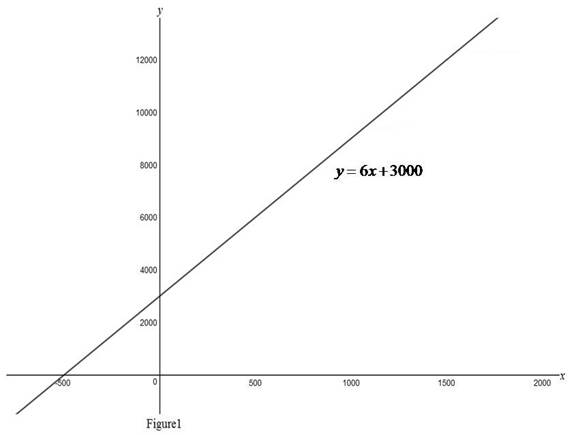# To express: The cost as a function of the number of toaster ovens produced.### Single Variable Calculus: Concepts...

4th Edition
James Stewart
Publisher: Cengage Learning
ISBN: 9781337687805### Single Variable Calculus: Concepts...

4th Edition
James Stewart
Publisher: Cengage Learning
ISBN: 9781337687805

#### Solutions

Chapter 1, Problem 22RE

(a)

To determine

## To express: The cost as a function of the number of toaster ovens produced.

Expert Solution

Solution:

The cost function in terms of the number of toaster ovens produced is y=6x+3000.

### Explanation of Solution

Given:

To produce 1000 toaster ovens in a week it costs $9000 and to produce 1500 it costs$12000.

The cost function follows a liner function.

Calculation:

Let the cost function as, yy1=m(xx1). (1)

The slope of the line m is given by m=yy1xx1.

According to the given data, there exist two points (1000,9000) and (1500,12000).

The slope of line passes through (1000,9000) and (1500,12000) is,

m=12000900015001000=6

Substitute m=6 in equation (1).

yy1=6(xx1)

Substitute (x,y)=(1000,9000) in yy1=6(xx1),

y9000=6(x1000)y9000=6x6000y=6x6000+9000y=6x+3000

Therefore, the cost function is, y=6x+3000.

Use online graphing calculator and obtain the graph of function y=6x+3000 as shown in Figure1.(b)

To determine

Expert Solution

### Explanation of Solution

From part (a), the slope of the line is, m=6 it represents the rate of change of cost with respect the number of production of toaster.

(c)

To determine

Expert Solution

### Explanation of Solution

From part (a), the equation is, y=6x+3000.

Notice that the y-intercept is \$3000. It represents the fixed cost even there is no production.

### Have a homework question?

Subscribe to bartleby learn! Ask subject matter experts 30 homework questions each month. Plus, you’ll have access to millions of step-by-step textbook answers!